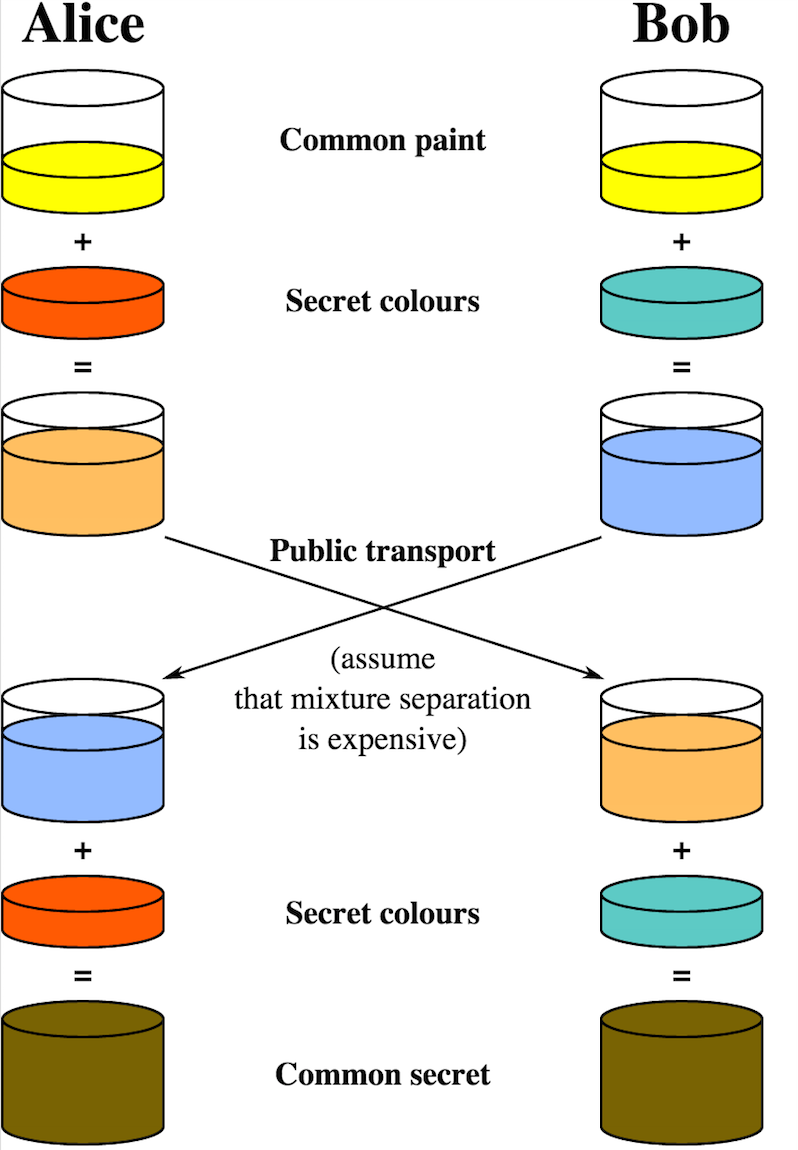# Deffie-Hellman 算法简介

Deffie-Hellman(简称 DH) 密钥交换是最早的密钥交换算法之一，它使得通信的双方能在非安全的信道中安全的交换密钥，用于加密后续的通信消息。 Whitfield Diffie 和 Martin Hellman 于 1976 提出该算法，之后被应用于安全领域，比如 Https 协议的 TSL(Transport Layer Security) 和 IPsec 协议的 IKE(Internet Key Exchange) 均以 DH 算法作为密钥交换算法。

# 数论知识

## 离散对数问题

``````{ a^1 mod p, a^2 mod p, ..., a^(p-1) mod p } = {1, 2, ... , p-1 }   {} 表示集合
``````

• a^x mod p 一定属于 {1, 2, …, p -1 }
• 如果 x != y，则 a^x mod p != a^y mod p
• 对于 1 <= b <= p - 1，一定存在唯一的 1 <= x <= p-1，使得 b = a^x mod p

## 求模公式

``````(a^x mod p)^y mod p = a^(xy) mod p
``````

``````令 a^x = mp + n， 其中 m, n 为自然数， 0 <= n < p，则有
C = (a^x mod p)^y mod p
= ((mp + n) mod p)^y mod p
= n^y mod p
= (mp +n)^y mod p
= a^(xy) mod p
``````

# Deffie-Hellman 算法原理

``````+-------------------------------------------------------------------+
|                    Global Pulic Elements                          |
|                                                                   |
|       p                               prime number                |
|       a                               prime number, a < p         |
+-------------------------------------------------------------------+
+-------------------------------------------------------------------+
|                    User A Key Generation                          |
|                                                                   |
|       Select private Xa               Xa < p                      |
|       Calculate public Ya             Ya = a^Xa mod p             |
+-------------------------------------------------------------------+
+-------------------------------------------------------------------+
|                    User B Key Generation                          |
|                                                                   |
|       Select private Xb               Xb < p                      |
|       Calculate public Yb             Yb = a^Xb mod p             |
+-------------------------------------------------------------------+
+-------------------------------------------------------------------+
|               Calculation of Secret Key by User A                 |
|                                                                   |
|       Secret Key K                    K = Yb^Xa mod p             |
+-------------------------------------------------------------------+
+-------------------------------------------------------------------+
|               Calculation of Secret Key by User B                 |
|                                                                   |
|       Secret Key K                    K = Ya^Xb mod p             |
+-------------------------------------------------------------------+
``````

``````K = Yb^Xa mod p
= (a^Xb mod p)^Xa mod p
= a^(Xa * Xb) mod p          根据上述求模公式
= (a^Xa mod p)^Xb mod p
= Ya^Xb mod p
``````

• 公开的数：a, p, Ya, Yb
• 非公开数：Xa, Xb, K